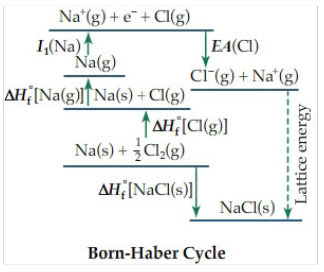# Problem: Rank the following ionic compounds by the magnitude of their lattice energy.Na2O, Na2S, MgO, BeO, LiClBy convention, the lattice energy is the energy required to form one mole of a solid ionic crystalline lattice from its gaseous ions. Since we cannot directly measure lattice energies, we generally use the Born-Haber cycle to calculate the lattice energy from other measurable quantities. For instance, the Born-Haber cycle of sodium chloride is shown below.In this cycle, the lattice energy is calculated using the following parameters. The associated reaction for each is shown in Figure 1.ΔH°f [NaCl(s)], enthalpy of formation of NaClΔH°f [Cl(g)], enthalpy of formation of gaseous ClΔH°f [Na(g)], enthalpy of formation of gaseous NaI1 (Na), first ionization energy of NaEA(Cl)], electron affinity of ClSimilar reactions and energy terms can be written to correspond to the Born-Haber cycle for the lattice energy of other ionic compounds

###### FREE Expert Solution

We are asked to rank the following ionic compounds by the magnitude of their lattice energy.

Na2O, Na2S, MgO, BeO, LiCl

The lattice energy can be estimated using:###### Problem Details

Rank the following ionic compounds by the magnitude of their lattice energy.

Na2O, Na2S, MgO, BeO, LiCl

By convention, the lattice energy is the energy required to form one mole of a solid ionic crystalline lattice from its gaseous ions. Since we cannot directly measure lattice energies, we generally use the Born-Haber cycle to calculate the lattice energy from other measurable quantities. For instance, the Born-Haber cycle of sodium chloride is shown below.

In this cycle, the lattice energy is calculated using the following parameters. The associated reaction for each is shown in Figure 1.

• ΔH°f [NaCl(s)], enthalpy of formation of NaCl
• ΔH°f [Cl(g)], enthalpy of formation of gaseous Cl
• ΔH°f [Na(g)], enthalpy of formation of gaseous Na
• I(Na), first ionization energy of Na
• EA(Cl)], electron affinity of ClSimilar reactions and energy terms can be written to correspond to the Born-Haber cycle for the lattice energy of other ionic compounds